Published: February 19, 2018, Edited by: Mads Hobye

# Wemos toturial: Basic input and output

This is a basic wemos tutorial with code examples for running different elements on the wemos:

## Detecting button pressed STATE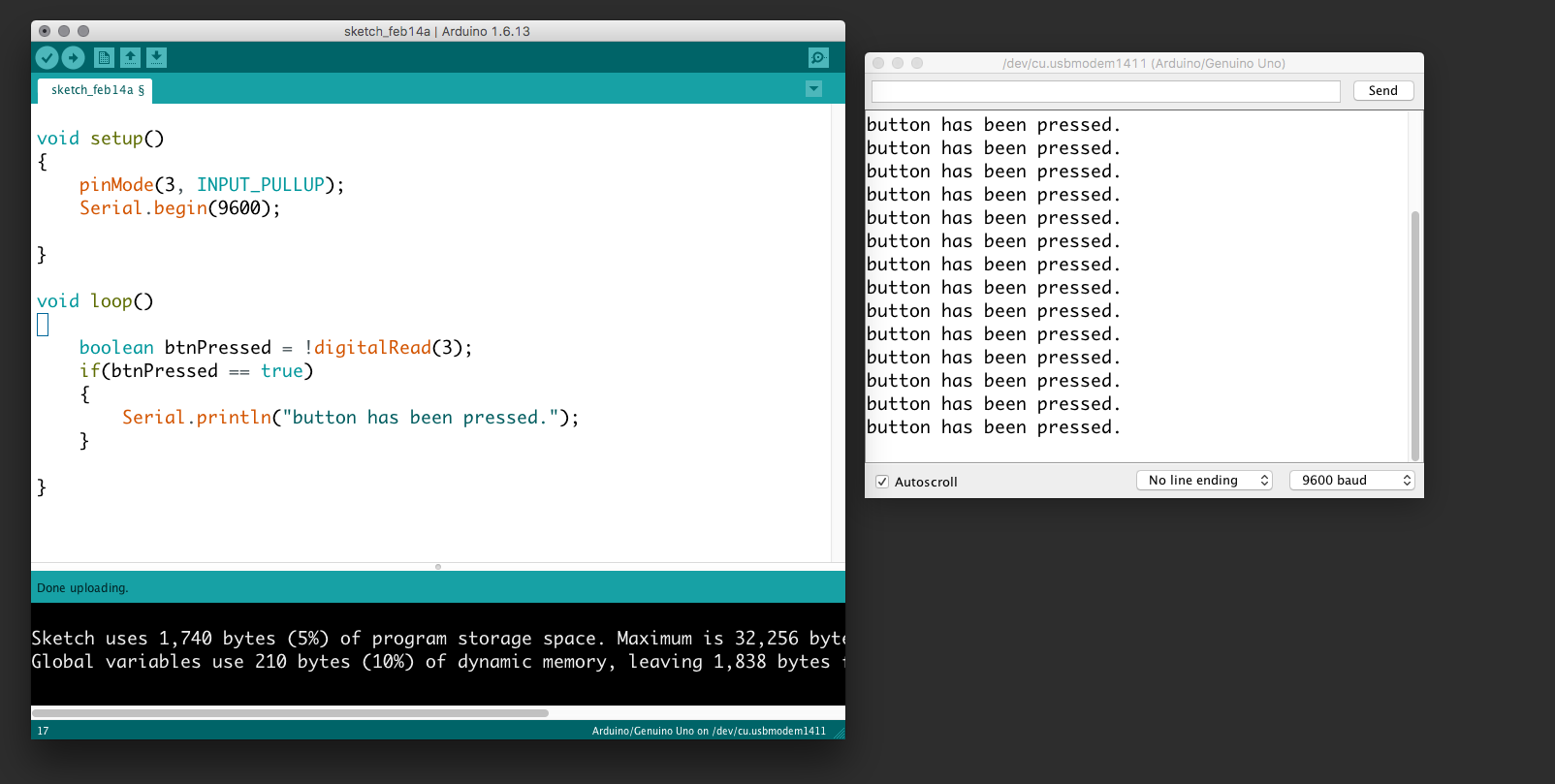In the following example, we connect a button to the Wemos and send a message repeatedly somebody presses it. Be aware that we use the internal pull-up so the button has to be connected to the ground. Further, we use the serial communication to receive the messages to our prompt. Use the "search icon" (upper right corner) to view the serial prompt.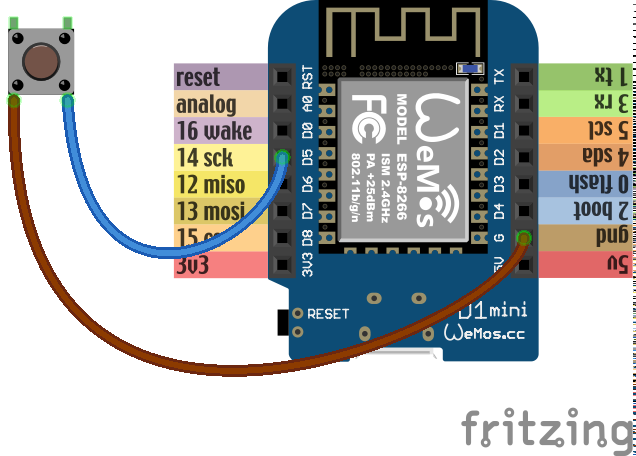``````        void setup()
{
pinMode(D5, INPUT_PULLUP);
Serial.begin(9600);

}

void loop()
{
if(btnPressed == true)
{
Serial.println("button has been pressed.");
}

}
``````

## Detecting button single press - An EVENT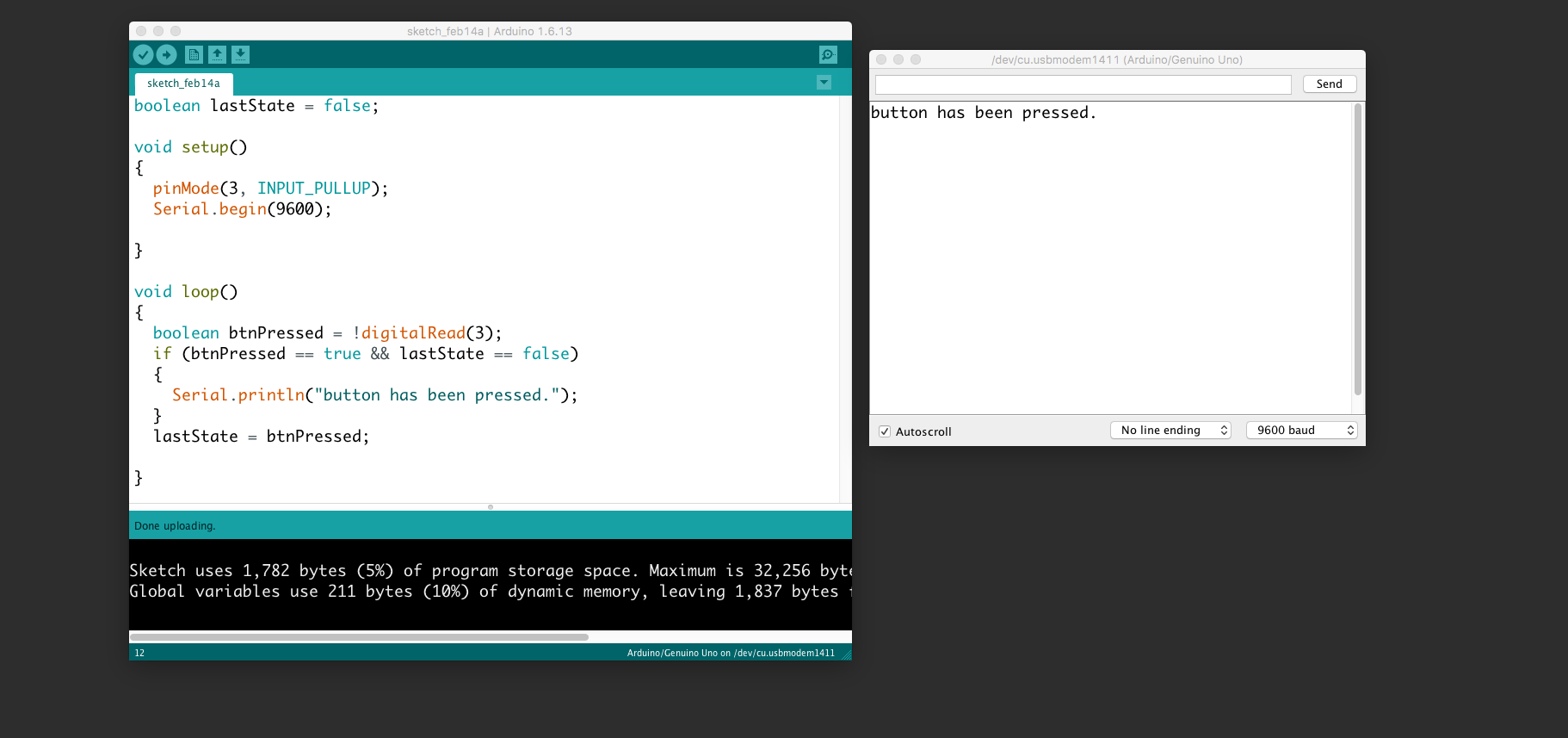The event of pressing the button. Use the same wiring as above.

``````  boolean lastState = false;

void setup()
{
pinMode(D5, INPUT_PULLUP);
Serial.begin(9600);

}

void loop()
{
if (btnPressed == true && lastState == false)
{
Serial.println("button has been pressed.");
}
lastState = btnPressed;

}
``````

## Control a led/relay etc.

To control a pin for a led or a relay etc.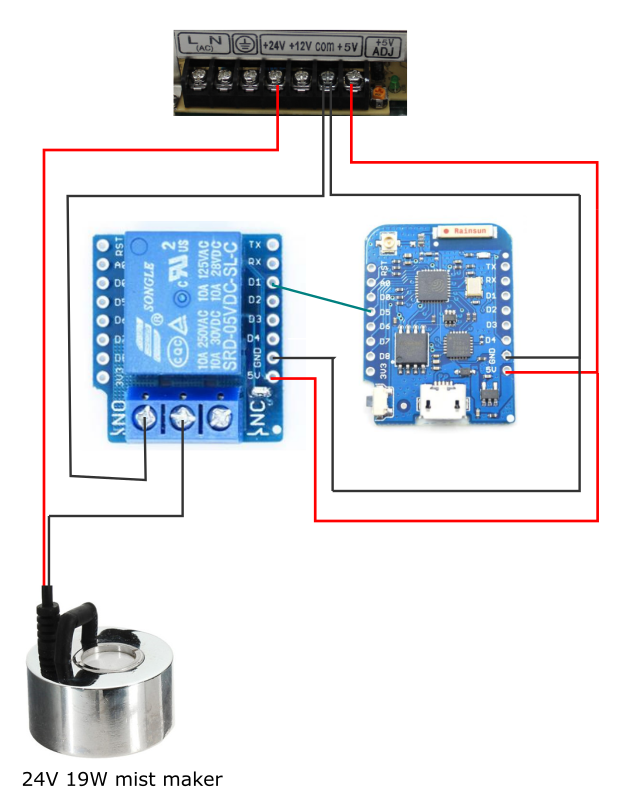``````    void setup() {

pinMode(D5, OUTPUT);
}

void loop() {
digitalWrite(D5, HIGH);
}
``````

## Control neopixels

Neopixels are versatile light strings where each diode can be controlled individually. Each one has Red, Green, and Blue and can be from 0 to 255 in brightness. Multiple libraries exists to use noepixels. We suggest you use fastled.Search for "fastled" and click install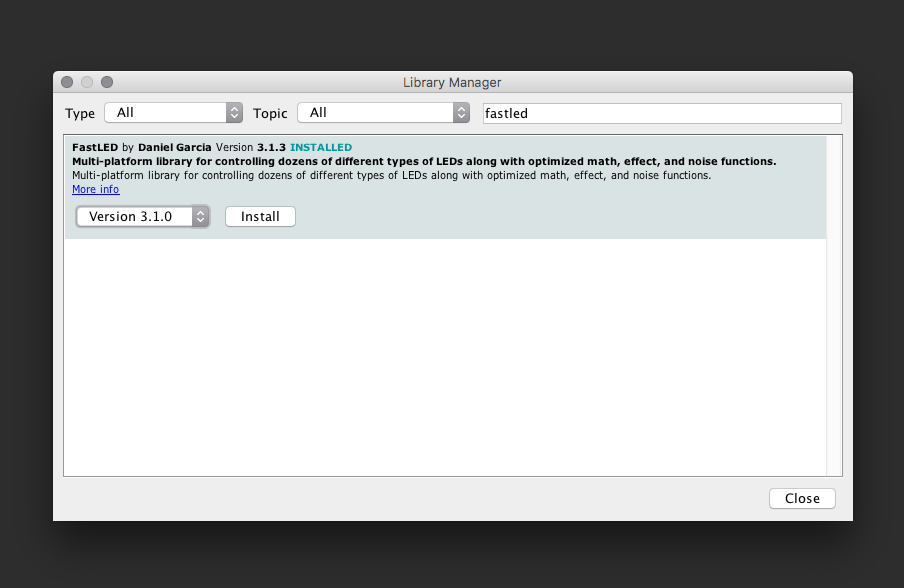Use this wiring: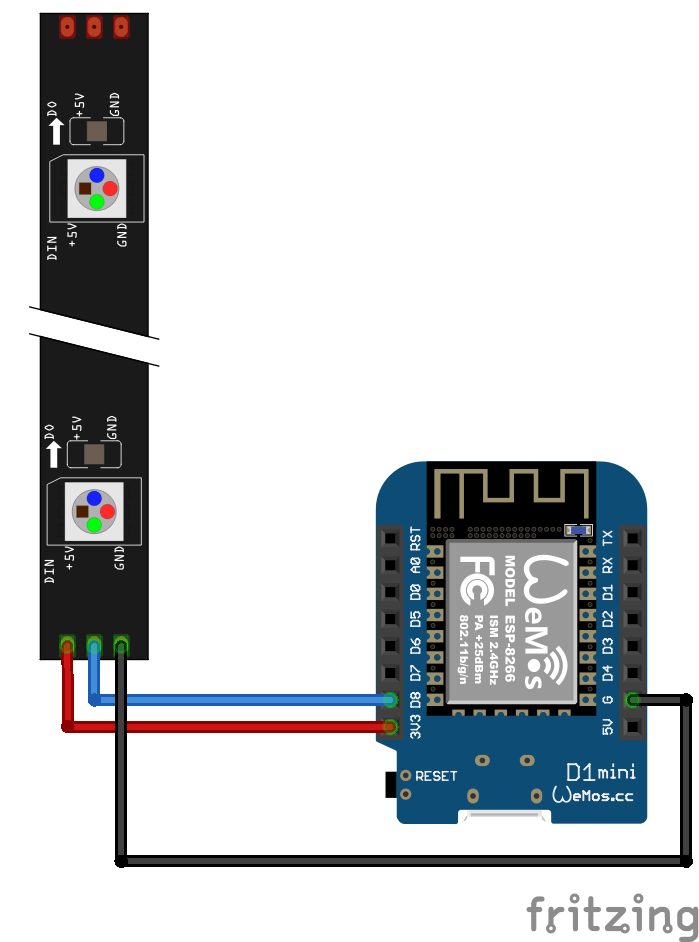IMPORTANT: connect 5v to v, Di to s and G to g AND make sure the direction is correct: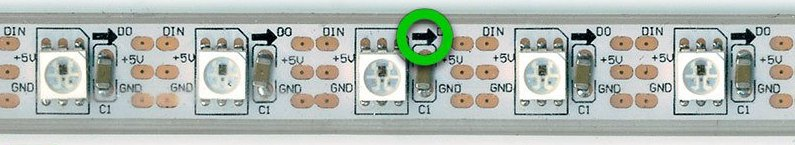This example sketch will turn red, green and blue for the first three pixels:

``````  #include "FastLED.h"

// How many leds in your strip?
#define NUM_LEDS 10
#define DATA_PIN 4

// Define the array of leds
CRGB leds[NUM_LEDS];

void setup() {
}

void loop() {
// Turn the LED on, then pause
leds= CRGB( 255, 0, 0);
leds= CRGB( 0, 255, 0);
leds= CRGB( 0, 0, 255);
FastLED.show();

}
``````

This example will turn all the 12 leds red:

``````#include "FastLED.h"

// How many leds in your strip?
#define NUM_LEDS 13
#define DATA_PIN D8

// Define the array of leds
CRGB leds[NUM_LEDS];

void setup() {
}

void loop() {

for (int i = 0; i < NUM_LEDS; i = i + 1)
{
leds[i] = CRGB( 255, 0, 0);
}
FastLED.show();

}
``````

## This example makes a chase

``````#include "FastLED.h"

// How many leds in your strip?
#define NUM_LEDS 13
#define DATA_PIN D8

// Define the array of leds
CRGB leds[NUM_LEDS];

void setup() {
Serial.begin(9600);
}
int numLedsOn = 0;
void loop() {

for (int i = 0; i < NUM_LEDS; i = i + 1)
{
if (i < numLedsOn)
{
leds[i] = CRGB( 255, 0, 0);
}
else
{
leds[i] = CRGB( 0, 0, 0);
}
}

FastLED.show();
numLedsOn = numLedsOn + 1;
if (numLedsOn == NUM_LEDS)
{
numLedsOn = 0;
}
Serial.println(numLedsOn);

delay(100);

}
``````

## Button + neopixels

(This has not been updated to Wemos - change pins accordingly)

This example connects the button (input) to the neopixels (output). Thus, your first interactive installation.``````  #include "FastLED.h"

// How many leds in your strip?
#define NUM_LEDS 10
#define DATA_PIN D8

// Define the array of leds
CRGB leds[NUM_LEDS];

void setup() {
pinMode(3, INPUT_PULLUP);
}

void loop() {

if (btnPressed == true)
{
leds = CRGB( 255, 0, 0);
leds = CRGB( 0, 255, 0);
leds = CRGB( 0, 0, 255);
}
else
{
leds = CRGB( 0, 0, 0);
leds = CRGB( 0, 0, 0);
leds = CRGB( 0, 0, 0);

}
FastLED.show();

}
``````

## Control a servo

Servos are motors in which their position can be controlled. They have an action radius of 0 to 180 degrees. Some servos are "hacked" to be continuous. They will rotate 360 degrees, but cannot be positioned. They are then able to move in a controlled speed and direction. Orange wire is signal and should be connected to S.``````  #include <Servo.h>

Servo myservo;  // create servo object to control a servo
// twelve servo objects can be created on most boards

int pos = 0;    // variable to store the servo position

void setup() {
myservo.attach(D0);  // attaches the servo on pin 9 to the servo object
}

void loop() {

for (pos = 0; pos <= 180; pos += 1) { // goes from 0 degrees to 180 degrees
// in steps of 1 degree
myservo.write(pos);              // tell servo to go to position in variable 'pos'
delay(15);                       // waits 15ms for the servo to reach the position
}
for (pos = 180; pos >= 0; pos -= 1) { // goes from 180 degrees to 0 degrees
myservo.write(pos);              // tell servo to go to position in variable 'pos'
delay(15);                       // waits 15ms for the servo to reach the position
}
}
``````

## Joystick

``````  void setup() {
Serial.begin(9600);
}

void loop() {
}
``````

## Joystick + servo

(This has not been updated to Wemos - change pins accordingly)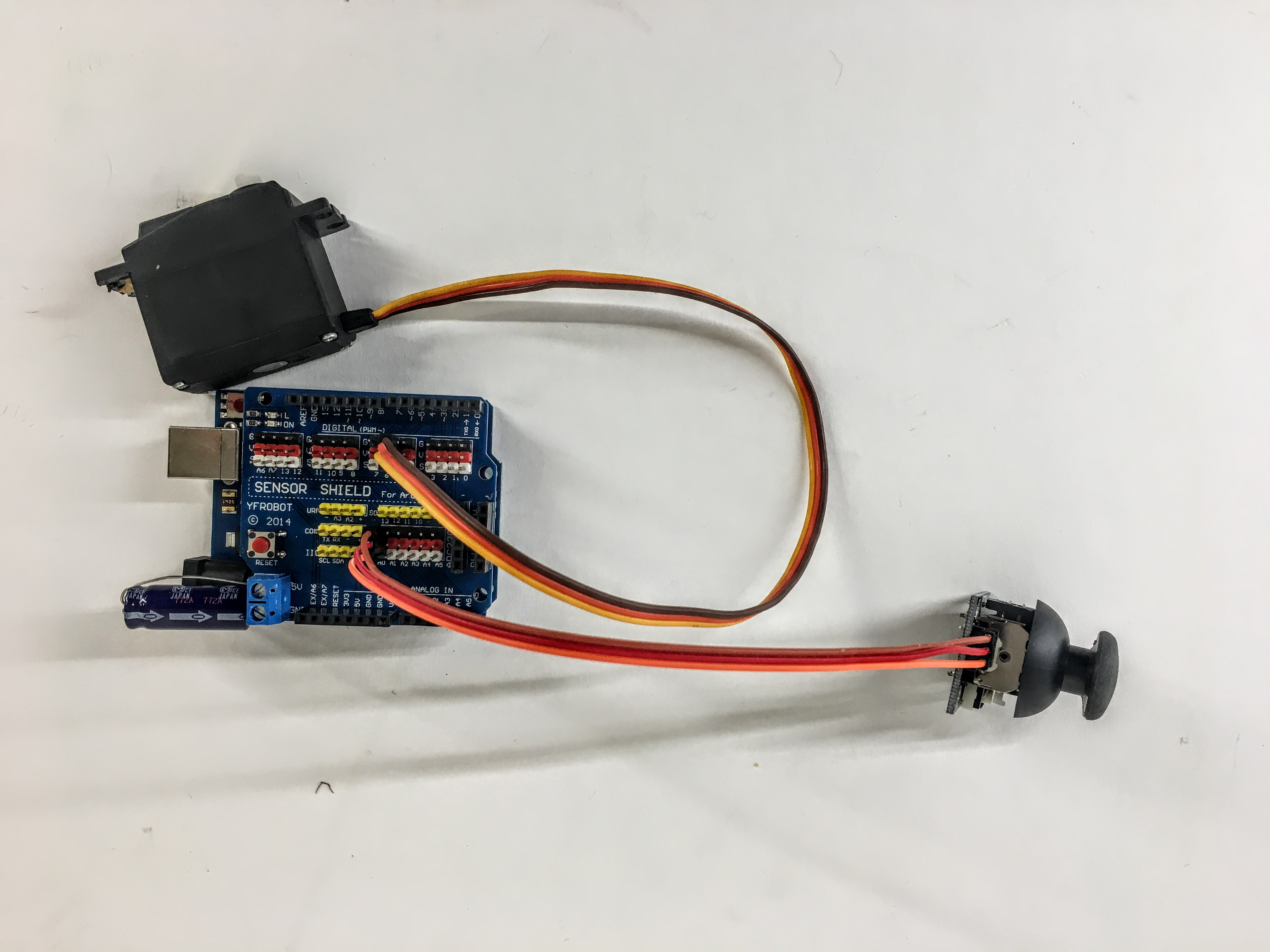``````    #include <Servo.h>

Servo myservo;

void setup() {
myservo.attach(D0);  // attaches the servo on pin D0 to the servo object
}

void loop() {

}
``````

## Distance sensor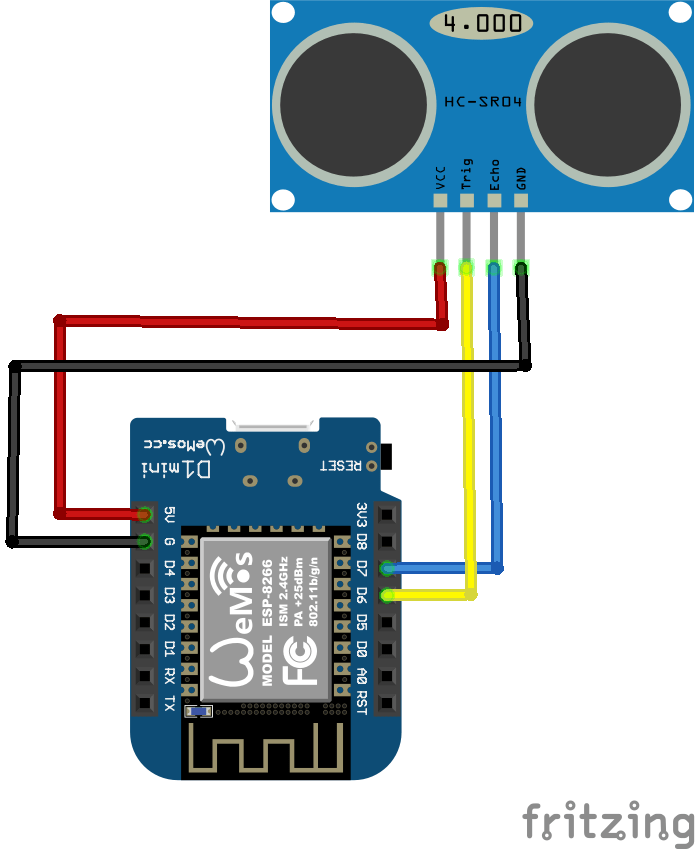One simple sensor to use is the distance sensor. This is an ultrasonic sensor which receives values from 0-1024 relative to about 15cm to 300cm.

``````    #define echoPin D7 // Echo Pin
#define trigPin D6 // Trigger Pin

long duration, distance; // Duration used to calculate distance

void setup()
{
Serial.begin (9600);
pinMode(trigPin, OUTPUT);
pinMode(echoPin, INPUT);
}

void loop()
{
/* The following trigPin/echoPin cycle is used to determine the
distance of the nearest object by bouncing soundwaves off of it. */
digitalWrite(trigPin, LOW);
delayMicroseconds(2);
digitalWrite(trigPin, HIGH);
delayMicroseconds(10);
digitalWrite(trigPin, LOW);
duration = pulseIn(echoPin, HIGH);
//Calculate the distance (in cm) based on the speed of sound.
distance = duration/58.2;
Serial.println(distance);
delay(50);
}
``````

## Timing without delay

Using delay is bad practice because it halts everything on the board. Instead use "millis()". This example does something every two seconds:

``````unsigned long timer = 0;
void setup()
{

}

void loop()
{
if(millis()-timer > 2000) // do something every two seconds)
{
timer = millis();
//do something here
}
}
``````

## MP3 Player

(This has not been updated to Wemos - change pins accordingly) Use the MP3 player to make interactive sounds for voice commands and games.Remember to install the library like above with neopixels: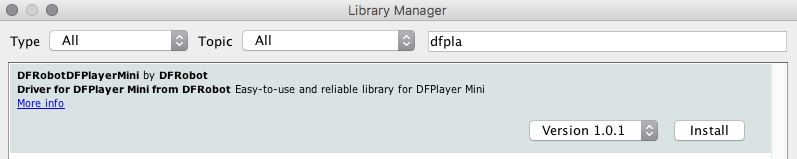This guide is the best so far

Simple sample code:

``````#include <SoftwareSerial.h>
#include "Arduino.h"
#include <DFRobotDFPlayerMini.h>

SoftwareSerial mySoftwareSerial(10, 11); // RX, TX - mp3
DFRobotDFPlayerMini myDFPlayer;
boolean lastState = false;

void printDetail(uint8_t type, int value);
void setup() {
mySoftwareSerial.begin(9600); //for mp3 player
Serial.begin(9600);

if (!myDFPlayer.begin(mySoftwareSerial)) { //Use softwareSerial to communicate with mp3.
Serial.println(F("Unable to begin:"));
while (true);
}
myDFPlayer.volume(20); //Set volume value. From 0 to 30

pinMode(3, INPUT_PULLUP);
}

void loop() {
if (btnPressed == true && lastState == false)
{
myDFPlayer.play(1); //Play the first mp3
Serial.println("button has been pressed.");
}
lastState = btnPressed;
}
``````

## Accelerometer

Use the accelerometer to detect movement.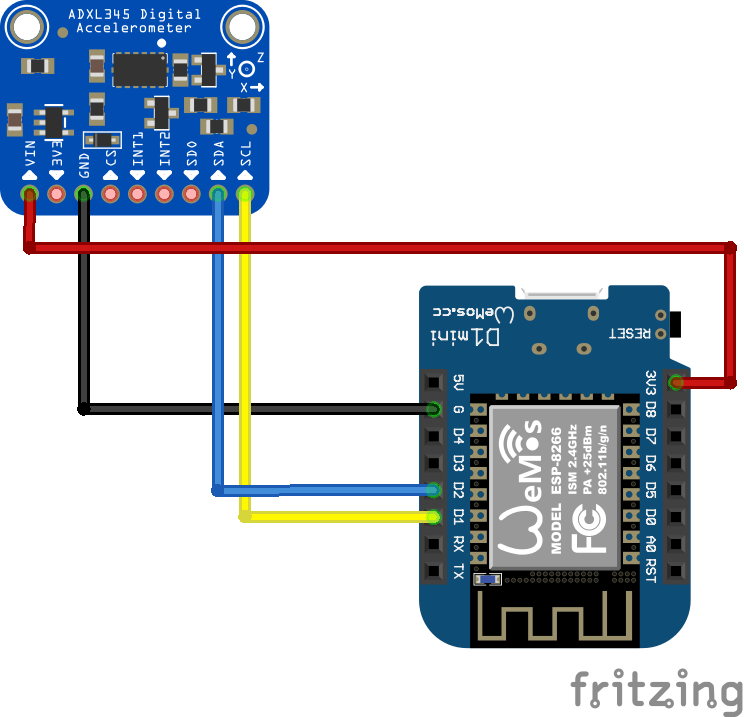``````    #include <Wire.h>

/* Assign a unique ID to this sensor at the same time */

void displaySensorDetails(void)
{
sensor_t sensor;
accel.getSensor(&sensor);
Serial.println("------------------------------------");
Serial.print ("Sensor: "); Serial.println(sensor.name);
Serial.print ("Driver Ver: "); Serial.println(sensor.version);
Serial.print ("Unique ID: "); Serial.println(sensor.sensor_id);
Serial.print ("Max Value: "); Serial.print(sensor.max_value); Serial.println(" m/s^2");
Serial.print ("Min Value: "); Serial.print(sensor.min_value); Serial.println(" m/s^2");
Serial.print ("Resolution: "); Serial.print(sensor.resolution); Serial.println(" m/s^2");
Serial.println("------------------------------------");
Serial.println("");
delay(500);
}

void displayDataRate(void)
{
Serial.print ("Data Rate: ");

switch(accel.getDataRate())
{
Serial.print ("3200 ");
break;
Serial.print ("1600 ");
break;
Serial.print ("800 ");
break;
Serial.print ("400 ");
break;
Serial.print ("200 ");
break;
Serial.print ("100 ");
break;
Serial.print ("50 ");
break;
Serial.print ("25 ");
break;
Serial.print ("12.5 ");
break;
Serial.print ("6.25 ");
break;
Serial.print ("3.13 ");
break;
Serial.print ("1.56 ");
break;
Serial.print ("0.78 ");
break;
Serial.print ("0.39 ");
break;
Serial.print ("0.20 ");
break;
Serial.print ("0.10 ");
break;
default:
Serial.print ("???? ");
break;
}
Serial.println(" Hz");
}

void displayRange(void)
{
Serial.print ("Range: +/- ");

switch(accel.getRange())
{
Serial.print ("16 ");
break;
Serial.print ("8 ");
break;
Serial.print ("4 ");
break;
Serial.print ("2 ");
break;
default:
Serial.print ("?? ");
break;
}
Serial.println(" g");
}

void setup(void)
{
Serial.begin(9600);
Serial.println("Accelerometer Test"); Serial.println("");

/* Initialise the sensor */
if(!accel.begin())
{
/* There was a problem detecting the ADXL345 ... check your connections */
while(1);
}

/* Set the range to whatever is appropriate for your project */

/* Display some basic information on this sensor */
displaySensorDetails();

/* Display additional settings (outside the scope of sensor_t) */
displayDataRate();
displayRange();
Serial.println("");
}

void loop(void)
{
/* Get a new sensor event */
sensors_event_t event;
accel.getEvent(&event);

/* Display the results (acceleration is measured in m/s^2) */
Serial.print("X: "); Serial.print(event.acceleration.x); Serial.print(" ");
Serial.print("Y: "); Serial.print(event.acceleration.y); Serial.print(" ");
Serial.print("Z: "); Serial.print(event.acceleration.z); Serial.print(" ");Serial.println("m/s^2 ");
delay(500);
}
``````